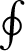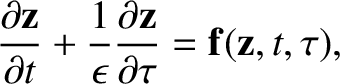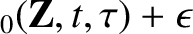Next: Invariance of Magnetic Moment Up: Charged Particle Motion Previous: Guiding Centre Motion

# Magnetic Drifts

Equations (70) and (86) can be combined to give(87)

The three terms on the right-hand side of the above expression are conventionally called the magnetic, or grad-B, drift, the inertial drift, and the polarization drift, respectively.

The magnetic drift,(88)

is caused by the slight variation of the gyroradius with gyrophase as a charged particle rotates in a non-uniform magnetic field. The gyroradius is reduced on the high-field side of the Larmor orbit, whereas it is increased on the low-field side. The net result is that the orbit does not quite close. In fact, the motion consists of the conventional gyration around the magnetic field combined with a slow drift which is perpendicular to both the local direction of the magnetic field and the local gradient of the field-strength.

Given that(89)

the inertial drift can be written(90)

In the important limit of stationary magnetic fields and weak electric fields, the above expression is dominated by the final term,(91)

which is called the curvature drift. As is easily demonstrated, the quantityis a vector whose direction is towards the centre of the circle which most closely approximates the magnetic field-line at a given point, and whose magnitude is the inverse of the radius of this circle. Thus, the centripetal acceleration imposed by the curvature of the magnetic field on a charged particle following a field-line gives rise to a slow drift which is perpendicular to both the local direction of the magnetic field and the direction to the local centre of curvature of the field.

The polarization drift,(92)

reduces to(93)

in the limit in which the magnetic field is stationary but the electric field varies in time. This expression can be understood as a polarization drift by considering what happens when we suddenly impose an electric field on a particle at rest. The particle initially accelerates in the direction of the electric field, but is then deflected by the magnetic force. Thereafter, the particle undergoes conventional gyromotion combined withdrift. The time between the switch-on of the field and the magnetic deflection is approximately. Note that there is no deflection if the electric field is directed parallel to the magnetic field, so this argument only applies to perpendicular electric fields. The initial displacement of the particle in the direction of the field is of order(94)

Note that, because, the displacement of the ions greatly exceeds that of the electrons. Thus, when an electric field is suddenly switched on in a plasma, there is an initial polarization of the plasma medium caused, predominately, by a displacement of the ions in the direction of the field. If the electric field, in fact, varies continuously in time, then there is a slow drift due to the constantly changing polarization of the plasma medium. This drift is essentially the time derivative of Eq. (94) [i.e., Eq. (93)].Next: Invariance of Magnetic Moment Up: Charged Particle Motion Previous: Guiding Centre Motion
Richard Fitzpatrick 2011-03-31